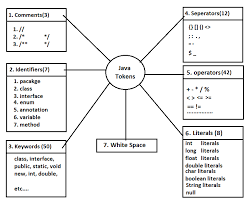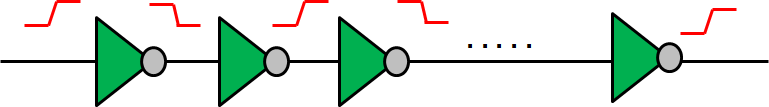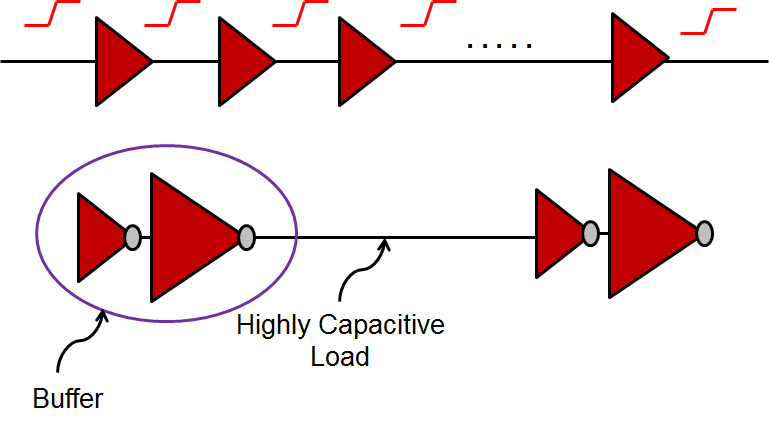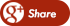﻿ Inverter vs Buffer based clock treeBack

## Inverter vs Buffer based clock treeA buffer is nothing but two inverters connected back to back. Does it make any difference if the CTS (Clock Tree Synthesis) is done using buffers or inverters? What exactly are the pros and cons and what factors would the backend design engineers take into account while deciding how to build their clock trees? I'm gonna answer these questions in this post.

An inverter based clock tree:

To keep things simple and pertinent to the discussion, let's assume that we are using only a single kind of inverter (i.e. of let's say drive X) to build our clock trees. And all the inverters are placed equidistant from each other. The scenario is shown in Fig. 1. Advantage of using an inverter based clock tree is that the high pulse width and the low pulse width would be symmetrical. For the clock signal, this is a critical requirement, especially for SoCs which have a high interaction between the positive and negative edge triggered flip-flops.Figure 1: Inverter Based Clock Tree giving equal rise and fall times

A buffer based clock tree:

While theoretically, one can create a buffer using two identical inverters connected back to back, that is generally not the way buffers are designed while designing the standard cell libraries. To save area, the first buffer is typically of a lower drive strength and is placed very closed to the second inverter. The second inverter, however, is of higher drive strength.Figure 2: Buffer Based Clock Tree. Buffer is formed by connecting two invertes back to back

One must also notice that the delay of first inverter is dominated by the load of the second inverter because the wire length between these two inverters is very small, hence one can neglect the wire cap. But for the second inverter, the load comprises of the wire cap as well as the input cap of the next buffer. This introduces an asymmetry in the rise and fall delays, and hence the high and low pulse widths of the clock signal.Figure 3: Difference in high and low pulse widths

For applications which have a very stringent requirement on the clock high and low pulse widths, one might prefer to use an inverter based clock tree over the buffer based clock tree.

Can we do something to make the buffer based clock tree work? The answer is yes! Let's take a look:

If we balance the load seen by first inverter and the load seen by the second inverter, we might be able to achieve equal rise and fall times, and hence equal high and low pulse widths for the clock transition signal.

In this approximation, we have modeled the wire in form of a T-model. And inverter is modeled using distributed RC model with it's "on" resistance and the diffusion capacitance.Figure 4: RC delay model for inverters and wire

To have the equal pulse widths for high and low times, the RC delay observed by the first inverter must be equal to the RC delay of the second inverter.

Rchn,1 (CD,1 + CG2) = Rchp,2 (CD,2 + Cwire + CG,1) + Rwire/2 (Cwire + CG,1) + Rwire/2 (CG,1)

If this equation is satisfied, one can say with a fair degree of confidence that the high and low pulse widths would be approximately equal. The resistance and capacitance of the wire is the function of its length and the same can be conveyed by the standard cell library designer to the backend designers.

While most standard cell library vendors provide a symmetrical buffer, there could well be a difference of a few pico-seconds in the buffer rise and fall delay, which creates a difference in the high and low pulse widths. The variation in the duty cycle increases for deeper clock trees!

A simple way to mitigate the problem is to insert an inverter in the middle point of the buffer-cased clock tree. The major challenge, however, lies in finding this middle point. This ensures that high and low pulse widths of the clock reaching at the sink pins of flip-flops is indeed the same!Figure 5: Inserting an inverter to maintain high and low pulse widths